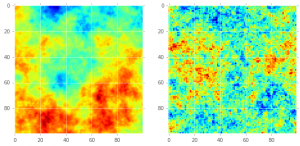# Stationary GMRF simulation with Discrete Fourier Transformation

### Algorithm to simulate GMRF with block-circulant Matrix.

Algorithm 2.10

Taken from: Rue, H., & Held, L. (2005). Gaussian Markov random fields: theory and applications. CRC press.

### let’s build the circulant matrix for the tourus

Oke, for the moment I´ll follow the example in GMRF book. i.e. a Torus (stationary of 128×128)

def createToroidalCircularBase(d=0.1,N=128):
"""
Creates a circular base similar to the one described in GMRF Rue and Held, 2005.
"""
c00 = np.hstack(((4 + d),-1,np.zeros(N - 3),-1))
c01 = np.hstack((-1,np.zeros(N - 1)))
c0 = np.zeros((N - 2 ,N))
c1 = np.vstack((c00,c01))
c = np.vstack((c1,c0))
c[N -1, 0] = -1
return c



%%time
## Create circular base
d = 0.00001
N = 100
c = createToroidalCircularBase(d=d,N=N)
## Simulate random noise (Normal distributed)
from scipy.fftpack import ifft2, fft2
zr = scipy.stats.norm.rvs(size=(c.size,2),loc=0,scale=1,random_state=1234)
zr.dtype=np.complex_
#plt.hist(zr.real)
#Lm = scipy.sqrt(C.shape*C.shape) * fft2(C)

Lm = fft2(c)
v = 1.0/ len(c) * fft2((Lm ** -0.5)* zr.reshape(Lm.shape))
x = v.real
plt.imshow(x,interpolation='None')
Both figures represent a simulation on a 100×100 cells. The comparison is as follows: on the right there is full gaussian field with mean zero and some Matern-based covariance structure. On the right a Markov Random Field simulation using the method described above.## [Bonus] Create a simple precision matrix

An easy way to create a precision matrix is with the function: *diags* inside the module: scipy.sparse. The code will look like this:

from scipy.sparse import diags
N = 10
diagonals = [np.zeros(N), np.ones(N-1), np.ones(N-1)]
# order 0 := diagonal , 1 first on top of diagonal, -1 first bottom of diagonal
offset = [0,1,-1]
## maps diagonals with position (offset)
M = diags(diagonals,offset).toarray()
## "seal the edges"
M = 1
M[N-1][N-1] =1
print(M)

Result:

[[ 1.  1.  0.  0.  0.  0.  0.  0.  0.  0.]
[ 1.  0.  1.  0.  0.  0.  0.  0.  0.  0.]
[ 0.  1.  0.  1.  0.  0.  0.  0.  0.  0.]
[ 0.  0.  1.  0.  1.  0.  0.  0.  0.  0.]
[ 0.  0.  0.  1.  0.  1.  0.  0.  0.  0.]
[ 0.  0.  0.  0.  1.  0.  1.  0.  0.  0.]
[ 0.  0.  0.  0.  0.  1.  0.  1.  0.  0.]
[ 0.  0.  0.  0.  0.  0.  1.  0.  1.  0.]
[ 0.  0.  0.  0.  0.  0.  0.  1.  0.  1.]
[ 0.  0.  0.  0.  0.  0.  0.  0.  1.  1.]]

## Update

There is a new tutorial for simulating a CAR model using a given adjacency matrix and spatial lattice. Go here to read it.### Juan Escamilla Mólgora

I'm a mathematical and computational statistical ecologist working at the intersection of Spatial Statistics, Software Development, Machine Learning and Cloud Computing. I'm researching novel methods for integration, harmonization and modelling of big environmental data. I developed the Wild Fire Alert and Monitoring System for Mexico and Central America

## 2 thoughts on “Stationary GMRF simulation with Discrete Fourier Transformation”

1.Juan says:

Worked, i tried the implementation.

Also, this is a test for comenting and moderating.

This site uses Akismet to reduce spam. Learn how your comment data is processed.